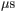# Problem: A proton is placed in a uniform electric field of 2950 N/C.A. Calculate the magnitude of the electric force felt by the proton.(F = ? )B. Calculate the proton's acceleration.( a= ? m/s2 )C. Calculate the proton's speed after 1.40  in the field, assuming it starts from rest.   ( V= ? m/s )

###### FREE Expert Solution

The electric force experienced by a proton in the uniform electric field:

$\overline{){\mathbf{F}}{\mathbf{=}}{\mathbf{q}}{\mathbf{E}}}$

Newton's second law:

$\overline{){\mathbf{F}}{\mathbf{=}}{\mathbf{m}}{\mathbf{a}}}$

We'll use the kinematic equation:###### Problem Details

A proton is placed in a uniform electric field of 2950 N/C.
A. Calculate the magnitude of the electric force felt by the proton.
(F = ? )
B. Calculate the proton's acceleration.
( a= ? m/s2 )

C. Calculate the proton's speed after 1.40in the field, assuming it starts from rest.

( V= ? m/s )